In the topic of Energy, students are often required to understand the factors affecting Gravitational Potential Energy and Kinetic Energy, as well as to answer open-ended questions on the different forms of energy.

Besides that, students are also required to state the energy conversion that occurs in an object/equipment in order for the equipment to function. Most students typically do not encounter much difficulties in such questions. The challenge usually arises when the students are asked to explain the reasons behind the energy conversion.

In this article, I will discuss the two main components of Energy Conversion:

Part 1: Stating Energy Conversions: How Does Energy Convert From One Form To Another

Part 2: Explaining The Reasons Behind Energy Conversions

## Background Information

Before I dive deeper into energy conversion, let us do a recap on the 6 key forms of Energy, which are:

• Potential Energy
• Gravitational Potential Energy
• Chemical Potential Energy
• Elastic Potential Energy
• Kinetic Energy
• Light Energy
• Heat Energy
• Electrical Energy
• Sound Energy

When we look at questions testing on the conversion of energy, we need to bear this important rule of energy in mind:

### “Energy cannot be created or destroyed. It can only be converted from one form to another.”

It means that the total energy (of any forms) in a system always remains the same.

## Part 1: Stating Energy Conversions: How Does Energy Convert From One Form To Another

Let us take a look at a few examples to gain a better understanding of energy conversion.

## Example 1: Torch

Before a torch can function, we need to place batteries in the torch. Why?

Batteries contain chemical potential energy in the batteries, which can be converted to electrical energy in the wires of the torch.

Lastly, the electrical energy in the wires is then converted to light energy in the bulb of the torch.

In summary, the main energy conversion in a torch is:## Example 2: A Ball Is Dropped From A Height To The Ground

At a height, the ball possesses gravitational potential energy.

Gravitational potential energy (GPE) is dependent on:

1) Height: The greater the height it is from the ground, the greater amount of GPE possessed by the object/person

2) Mass: The greater the mass of the object/person, the greater amount of GPE possessed by the object/person

When the ball is falling towards the ground, the gravitational potential energy that it possessed is gradually converted to kinetic energy (moving energy). Thus, as the ball falls, the amount of gravitational potential energy decreases while the amount of kinetic energy increases. As the ball is falling midway, it possesses both kinetic energy and gravitational potential energy.

When the ball hits the ground, there is friction between the surface of the ball and the ground, causing heat and sound to be produced. Hence, the kinetic energy of the ball is converted to heat energy and sound energy to overcome friction between the surface of the ball and the ground.

In summary, the main energy conversion in a falling ball is:With this information, let us take a look at the question below!

## Here’s A Question: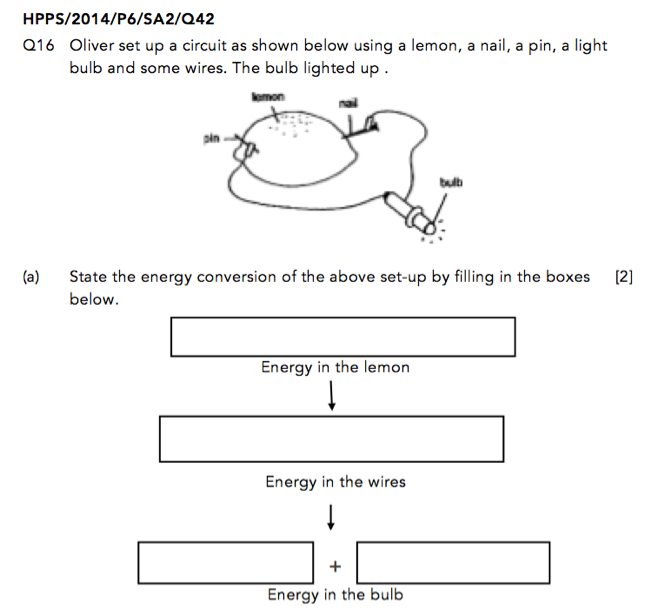Source: Henry Park Primary School – 2014 P6 Science Preliminary Examination Paper [Q42]

In this question, an electrical circuit was created using a lemon, a nail, some wires, and a light bulb.

Lemon is a type of food and possesses chemical potential energy.

The chemical potential energy in the lemon will then be converted to electrical energy in the wires.

The electrical energy in the wires will then be converted to light energy in the bulb, causing it to light up. In the process, the bulb will also feel hot as some of the electrical energy is converted to heat energy.

NOTE: The function of a bulb is to produce light. Thus, light energy must be written before heat energy.While stating the energy conversion in an object, many students tend to get confused between the “+” symbol and the “→” symbol.

Does your child when to use the “+” symbol and the “→” symbol?

## The Use Of Symbols: “+” & “→”

We use the “+” symbol when the two types of energy are occurring at the same time.

On the other hand, we use the “→” symbol when one type of energy is converted to another type of energy or transferred to another object.

Let us look at the energy conversion in a TV in order to better understand the above definitions.

## Energy Conversions In A Television Set

When we turn on the switch, electricity would flow from the electrical mains to the wires in the TV. The TV is now turned on and we will be able to see images on the TV screen and hear sounds from the speakers at the same time.

Since the source of energy is from the electrical mains, the first type of energy would be electrical energy from the electrical mains. This electrical energy will then be transferred to the wires in the TV. Thus, we use the symbol “→” to illustrate that.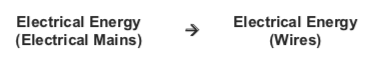The electrical energy in the wires would then be converted to BOTH light energy on the screen so we can see the images and sound energy in the speakers so we can enjoy our television programme. As such, we use a “+” symbol for light energy and heat energy.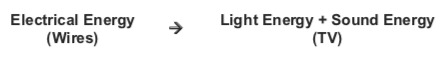Thus, the full conversion of energy in a TV would be: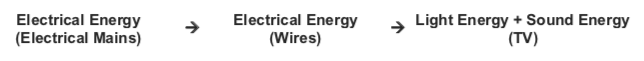## Part 2: Explaining The Reasons Behind Energy Conversions

In this section, I’ll sharing the reasons behind certain energy conversions, with reference to the following questions.

## Here’s A Question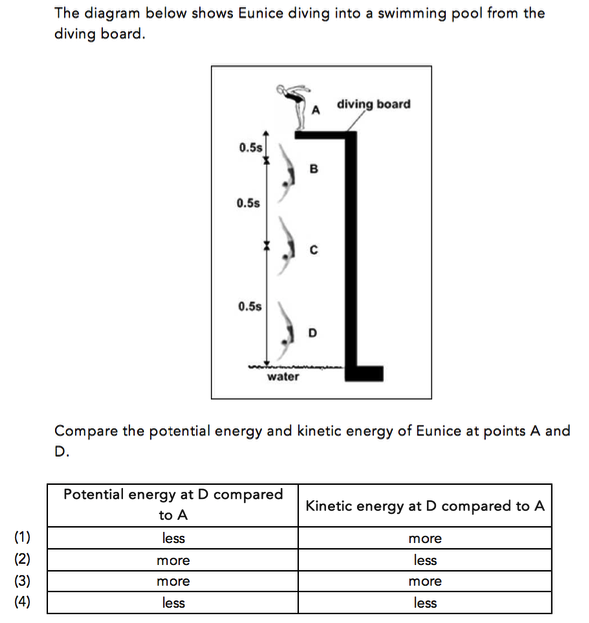Source: Beacon Primary School – 2014 P6 Preliminary Examination Paper [Q2]

## To Tackle The Above Question:

This question requires students to compare the GPE and KE at Points A and D.

GPE at Points A and D: Eunice is at a greater height at Point A than Point D. Hence, the GPE possessed at Point A is greater than the GPE possessed at Point D. This means that the GPE at Point D is lesser than the GPE possessed at Point A.

KE at Point A and D: At Point D, most of the GPE of Eunice at Point A has been converted to KE at Point D. Hence the KE at Point D will be greater than the KE at Point A.

Thus, the answer for this question would be Option 1.

## Let’s Have A Look At Another Question: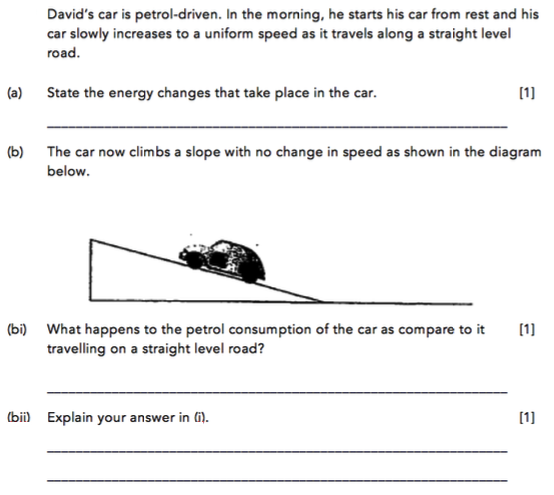Source: Methodist Girls’ School (Primary) – 2012 P6 SA1 Science Examination Paper [Q43]

## How We Tackle The Above Question:

For Part (A)

The petrol in the car possesses chemical potential energy, which is then converted to kinetic energy of the car when it moves.

Thus, the answer to this part is:

Chemical Potential Energy → Kinetic Energy

Most students would find this part relatively easy!

For Part (B)

The car now moves up the slope with the same speed.

The term, “same speed“, implies that the speed of the car remains constant and will not affect the energy changes.

As compared to travelling on a straight road, driving up a slope will cause the car to gain gravitational potential energy. This would mean that more chemical potential energy is now needed to convert into more kinetic energy as some of the kinetic energy is required to convert into gravitational potential energy.

Hence, the answer for part b(i) would be:

The petrol consumption of the car increases.

As for the explanation for part b(ii), it would be:

When the car travels up a slope, more chemical potential energy from the petrol is required to be converted to more kinetic energy to travel the same distance. This is because some of the kinetic energy of the car is converted to gravitational potential energy as the car moves up the slope unlike travelling on a straight road. Hence, more petrol is needed to drive up a slope as compared to moving on a straight road.

## In Conclusion

I hope this article has provided a comprehensive guide to the energy conversion in an object/equipment. Now, you should be able to better differentiate when to use the “+” symbol and the “→” symbol, and also to provide explanations behind energy conversions.

Stay tuned for more articles!If you like our methodology, we’ve some upcoming workshops: